# Pollution control

POLLUTION CONTROL COURSEWORK

An electrochemical reactor is used, in the batch mode, for the removal of Cadmium (initial concentration 1000 ppm) from a sulphuric acid solution, using insoluble, platinised titanium anodes. The electrolyte volume within the reactor is 20dm3 and the reactor operates at a current of 20A.

Species present in the electrolyte: Cd2+, H2O, H+, SO42-

Z= Number of electrons = 2

V = Reactor volume (20 dm3)

a)

The commonest basic design for an electrochemical cell is as shown below:

The components of the system, which make electrical contact with the oxidant and reductant solutions, are the electrodes. The electrode at which oxidation occurs, i.e., the electrode in contact with the reductant solution, is known as the anode. The electrode at which reduction occurs, i.e., the electrode in contact with the oxidant solution, is known as the cathode.

The reactions occurring in this example are:

CATHODE:

Cadmium deposition : Cd2+ + 2e- ! Cd!

Hydrogen evolution: 2H+ + 2e- ! H2

ANODE:

Oxygen evolution: H2O - 4e- ! O2 + 4H+

b)

It is now required to calculate the minimum time required to remove all metal from solution. The minimum amount of Cadmium to be removed may be estimated from the initial amount of Cadmium in the solution (Co).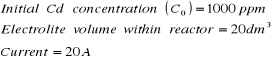It is known that:

In order to operate without unit errors, I will transform all these parameters into the International System of Units. Therefore:

And therefore:

The Faraday constant, and the molar mass of Cadmium are also known variables.

Under constant current conditions, Faraday's laws may be expressed as:

Where:

Equation 1 may be arranged to obtain the time:

The only unknown variable in equation 2 is n, the amount of material, or number of moles of Cadmium to be removed from the solution.

n can be obtained as the ratio between the mass of the Cadmium present in the electrolyte, and its molecular mass.

With the initial concentration of Cadmium (C0) and the volume of the electrolyte, the mass of Cadmium can be calculated:

Knowing that M=112.4 g/mol, then:

Equation 2 can now be solved:

c)

The overall limiting current of the reactor is calculated here knowing that:

• Averaged mass transport coefficient, Km = 2x10-3 cm/s (2x10-5 m/s)

• Number of cathodes = 30

• Area of single cathode = 160 cm3

• Therefore, total area = 30x160cm3 = 4800cm3 (0.28m3)

In mass transport control, the maximum current for the reaction is equal to the limiting current IL.

Where: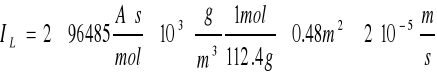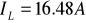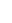d)

Calculation of the percentage current efficiency ()=IL/ITotal = (6.48/20) x100

=82.5%

e)

Batch time required to reach 1ppm Cadmium concentration.

The decay constant for the reaction (k) may be obtained from the following: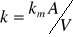Under mass transport controlled conditions, the decay may be represented by the following exponential relationship: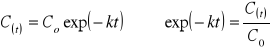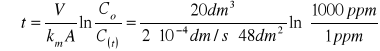t=14391.15s " 4 hrsf) Methods for improving the performance of the batch reactor.

Improving the performance of the reactor means decreasing its batch time, and achieving a higher metal purity. There are several ways in which these two points may be achieved:

Mass transfer may be increased with a better agitation in the system. This increase in mass transfer will therefore reduce the batch time.

Rotating cylinder cell types provide very high mass transport, therefore its use will reduce the batch time.

Rotating cylinder cell types also improve the metal purity by direct reduction of metal into powder, which is then easily removed from the solution.

The batch time may also be reduce with temperature. Following Arrhenius relationship, an increase in temperature will increase the rate constant, thus reducing the batch time.

Operating at a limiting current (of 16.48 A) will stop the hydrogen reaction from occurring, and the purity of the metal will become higher.

Dilution of the feed. If the feed is diluted (sulphuric acid may be diluted with water), the initial concentration of Cadmium will be decreased, which will lead to an overall decrease in the batch time.

Neutralising the sulphuric solution with a base such as sodium hydroxide may also reduce hydrogen production. In this case, H+ ions will react with OH- to form water, and so the production of Hydrogen in the cathode will be reduced. However, this method has other effects, like the formation of a cadmium base, and salt deposition, which will need other treatments.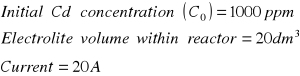Equation 1.Equation 2.The time required to remove all metal ion from solution is 28.62 minutesEnviado por: Superly Idioma: inglés País: España

Palabras clave:
Te va a interesar